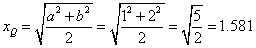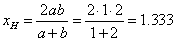## Relationship between Means

Here are some relationship between the means or averages ( Bronshtein et al, 2003 )

• If the data is all positive, the arithmetic mean is always greater than or equal to the geometric mean of the same data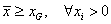, or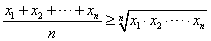• For any real data, quadratic mean is always greater or equal to absolute value of arithmetic mean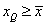, or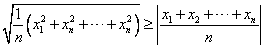• Special for only two positive numbers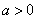and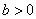, we have

Arithmetic mean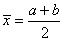Geometric mean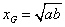Quadratic mean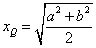Harmonic meanIf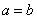, then all the means are equal: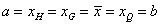If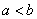, then the order is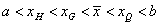Example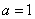,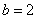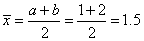,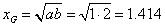,